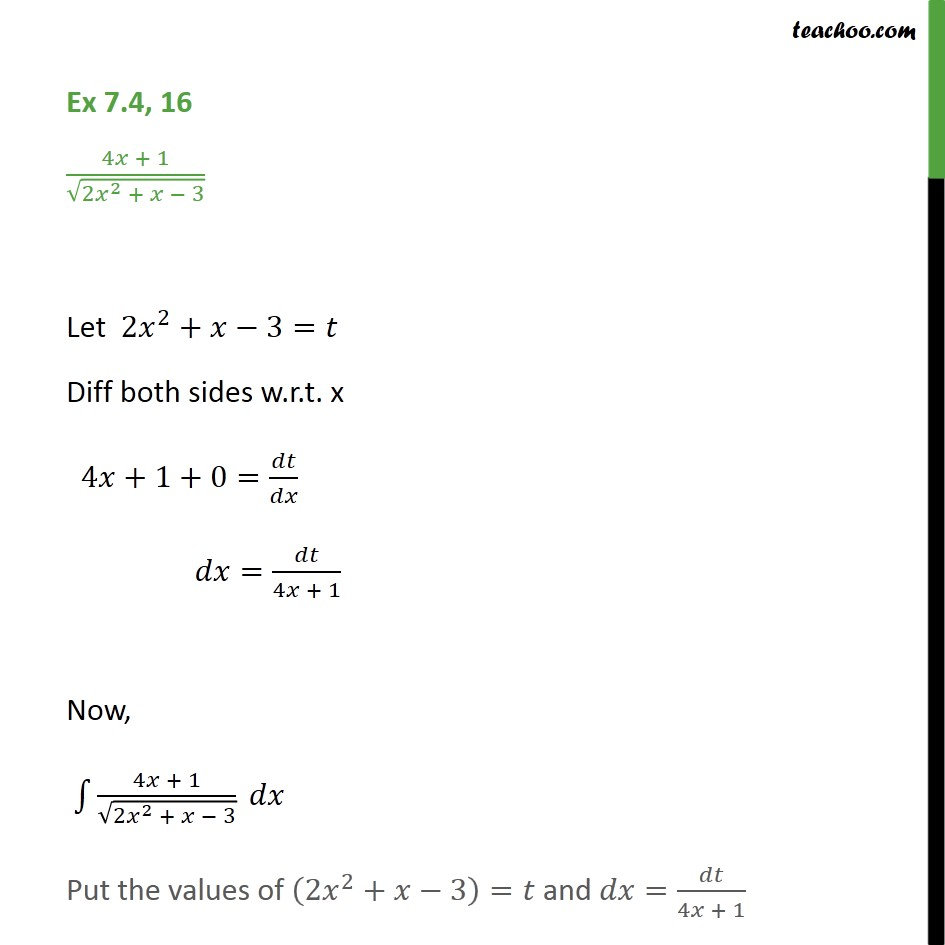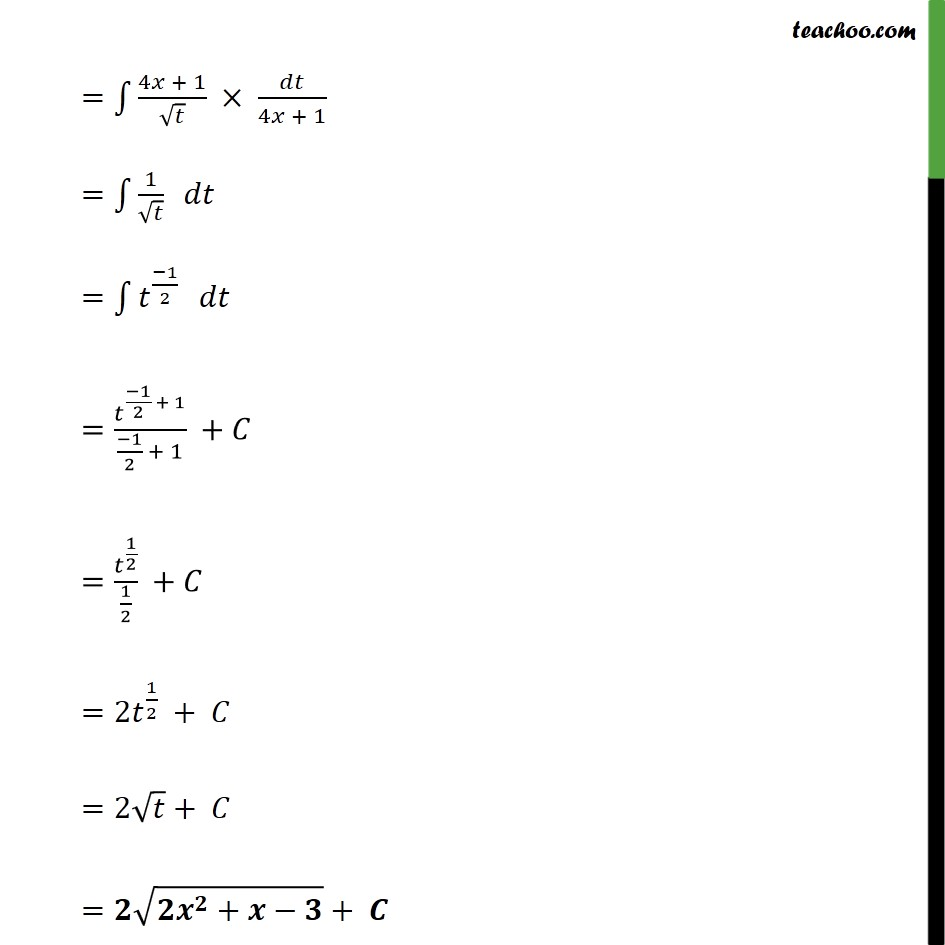Integration by specific formulaes - Method 10

Chapter 7 Class 12 Integrals
Concept wiseLearn in your speed, with individual attention - Teachoo Maths 1-on-1 Class

### Transcript

Ex 7.4, 16 (4 + 1)/ (2 ^2 + 3) Let 2 ^2+ 3= Diff both sides w.r.t. x 4 +1+0= / = /(4 + 1) Now, 1 (4 + 1)/ (2 ^2 + 3) Put the values of (2 ^2+ 3)= and = /(4 + 1) = 1 (4 + 1)/ /(4 + 1) = 1 1/ = 1 ^(( 1)/2) = ^(( 1)/2 + 1)/(( 1)/2 + 1) + = ^(1/2)/(1/2) + =2 ^(1/2) + =2 + = ( ^ + )+